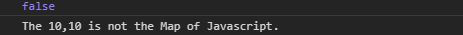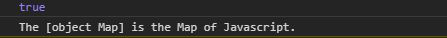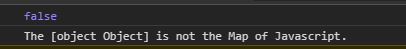# Underscore.js _.isMap() Function

• Last Updated : 25 Nov, 2021

Underscore.js is a library in javascript that makes operations on arrays, string, objects much easier and handy. _.isMap() function is used to check whether the given object is javascript Map or not.

Note: It is very necessary to link the underscore CDN before going and using underscore functions in the browser. When linking the underscore.js CDN The “_” is attached to the browser as a global variable.

Syntax:

```_.isMap(object);
```

Parameters :

• object : It is any javascript object such as array, string, maps, set etc.

Returns : It returns the boolean value. If the object is a Map of javascript it returns true otherwise false is returned by the function.

Few Examples are given below for a better understanding of the function.

Example 1:

When an array is given the output is false.

 ```<``html` `lang``=``"en"``>``<``head``>``  ``<``meta` `charMap``=``"UTF-8"``>``  ``<``meta` `name``=``"viewport"` `        ``content``=``"width=device-width, initial-scale=1.0"``>``  ``<``title``>Document``  ``<``script` `src``=``"https://cdnjs.cloudflare.com/ajax/libs/underscore.js/1.9.1/underscore-min.js"` `> ``  `` ````<``body``>``  ``<``script``>``    ``//creating a array of size 2 using constructor``    ``var obj= new Array(2);``    ``//filling array with value 10``    ``obj.fill(10);``    ``//using the underscore.js function _.isMap()``    ``var isMap= _.isMap(obj);``    ``console.log(isMap)``    ``//If the given object is Map it prints the object is Map.``    ``if(isMap)``    ``console.log(`The \${obj} is the Map of Javascript.`)``    ``else``    ``console.log(`The \${obj} is not the Map of Javascript.`)``  ```````

Output:Example 2:

When a Map is given it returns true.

 ```<``html` `lang``=``"en"``>``<``head``>``  ``<``meta` `charMap``=``"UTF-8"``>``  ``<``meta` `name``=``"viewport"` `        ``content``=``"width=device-width, initial-scale=1.0"``>``  ``<``title``>Document``  ``<``script` `src``=``"https://cdnjs.cloudflare.com/ajax/libs/underscore.js/1.9.1/underscore-min.js"` `> ``  `` ````<``body``>``  ``<``script``>``    ``//creating a Map using constructor``    ``var obj= new Map();``    ``//using the underscore.js function _.isMap()``    ``var isMap= _.isMap(obj);``    ``console.log(isMap)``    ``//If the given object is Map it prints the object is Map.``    ``if(isMap)``    ``console.log(`The \${obj} is the Map of Javascript.`)``    ``else``    ``console.log(`The \${obj} is not the Map of Javascript.`)``  ```````

Output:Example 3:

When giving an object as a parameter the output is false

 ```<``html` `lang``=``"en"``>``<``head``>``  ``<``meta` `charMap``=``"UTF-8"``>``  ``<``meta` `name``=``"viewport"` `        ``content``=``"width=device-width, initial-scale=1.0"``>``  ``<``title``>Document``  ``<``script` `src``=``"https://cdnjs.cloudflare.com/ajax/libs/underscore.js/1.9.1/underscore-min.js"` `> ``  `` ````<``body``>``  ``<``script``>``    ``//creating a Javascript Object using constructor``    ``var obj= new Object();``    ``obj={``      ``"a":1,``      ``"b":2``    ``}``    ``//using the underscore.js function _.isMap()``    ``var isMap= _.isMap(obj);``    ``console.log(isMap)``    ``//If the given object is Map it prints the object is Map.``    ``if(isMap)``    ``console.log(`The \${obj} is the Map of Javascript.`)``    ``else``    ``console.log(`The \${obj} is not the Map of Javascript.`)``  ```````

Output:My Personal Notes arrow_drop_up